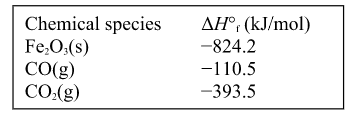# Problem: Calculate the enthalpy change (ΔH°rxn ) for the following reaction at 25°C. The value of ΔH°f in kJ/mol is given below.  2 Fe2O3(s) + 6 CO(g) → 4 Fe(s) + 6 CO2(g) A. −1380 kJ B. −49.6 kJ C. 541 kJ D. −3350 kJ E. −24.8 kJ

###### Problem Details

Calculate the enthalpy change (ΔH°rxn ) for the following reaction at 25°C. The value of ΔH°f in kJ/mol is given below.

2 Fe2O3(s) + 6 CO(g) → 4 Fe(s) + 6 CO2(g)

A. −1380 kJ

B. −49.6 kJ

C. 541 kJ

D. −3350 kJ

E. −24.8 kJWhat scientific concept do you need to know in order to solve this problem?

Our tutors have indicated that to solve this problem you will need to apply the Enthalpy of Formation concept. You can view video lessons to learn Enthalpy of Formation. Or if you need more Enthalpy of Formation practice, you can also practice Enthalpy of Formation practice problems.

What is the difficulty of this problem?

Our tutors rated the difficulty ofCalculate the enthalpy change (ΔH°rxn ) for the following re...as low difficulty.

How long does this problem take to solve?

Our expert Chemistry tutor, Jules took 2 minutes and 12 seconds to solve this problem. You can follow their steps in the video explanation above.

What professor is this problem relevant for?

Based on our data, we think this problem is relevant for Professor Mong's class at PITT.